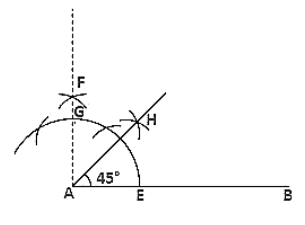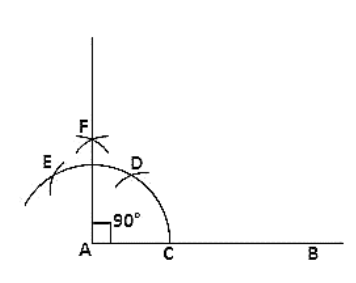# Construct the following angles at the initial point of a given ray and justify the construction:

Question:

Construct the following angles at the initial point of a given ray and justify the construction:

(i) 45°

(ii) 90°

Solution:

(i) Steps of construction:

1. Draw a line segment AB and produce BA to C.

2. Keeping A as the center and any radius draw an arc which intersects AC at D and AB at E.

3. Keeping D and E as center and radius more than half of DE draw arcs which intersect each other at F.

4. Join FA which intersects the arc in (2) at G.

5. Keeping G and E as center and radius more than half of GE draw arcs which intersect each other at H.

6. Join HA.

Therefore ∠HAB = 45°(ii) Steps of construction

1. Draw a line segment AB.

2. Keeping A as the center and any radius draw an arc which intersects AB at C.

3. Keeping C as center and the same radius draw an arc which intersects the previous arc at D.

4. Keeping D as the center and same radius draw an arc which intersects arc in (2) at E.

5. Keeping E and D as center and radius more than half of ED draw arcs which intersect each other at F.

6. Join FA.

Therefore ∠FAB = 90°